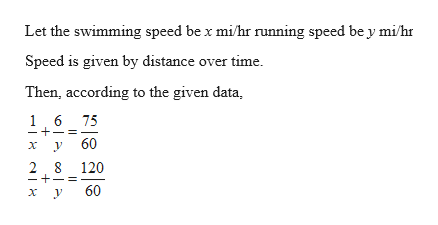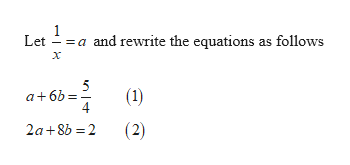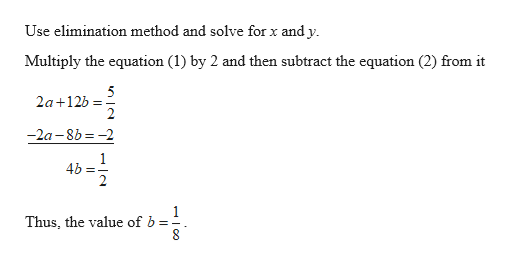# Shelia swam 1 mi and ran 6 mi in a total of 1 hr 15 min . In another training session she swam 2 mi and ran 8 mi in a total of 2 hr. Determine the speed at which she swims and the speed at which she runs. Assume that her swimming speed was the same each day and that her running speed was the same each day.

Question
1 views

Shelia swam 1 mi and ran 6 mi in a total of 1 hr 15 min . In another training session she swam 2 mi and ran 8 mi in a total of 2 hr. Determine the speed at which she swims and the speed at which she runs. Assume that her swimming speed was the same each day and that her running speed was the same each day.

check_circle

Step 1help_outlineImage TranscriptioncloseLet the swimming speed be x mi/hr running speed be y mi/hr Speed is given by distance over time. Then, according to the given data, 6 75 1. 60 2. 8 120 60 || fullscreen
Step 2help_outlineImage Transcriptionclose= a Let and rewrite the equations as follows 5 a+6b: 4 (1) (2) 2a+8b = 2 fullscreen
Step 3help_outlineImage TranscriptioncloseUse elimination method and solve for x and y. Multiply the equation (1) by 2 and then subtract the equation (2) from it 5 2a+12b = -2a -8b = -2 4b =; Thus, the value of b fullscreen

### Want to see the full answer?

See Solution

#### Want to see this answer and more?

Solutions are written by subject experts who are available 24/7. Questions are typically answered within 1 hour.*

See Solution
*Response times may vary by subject and question.
Tagged in
MathAlgebra

### Equations and In-equations